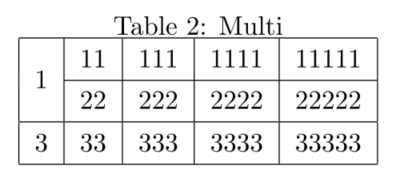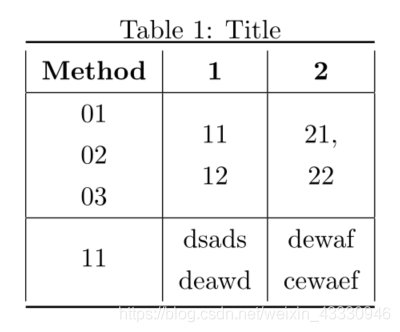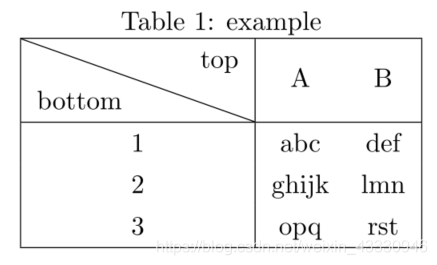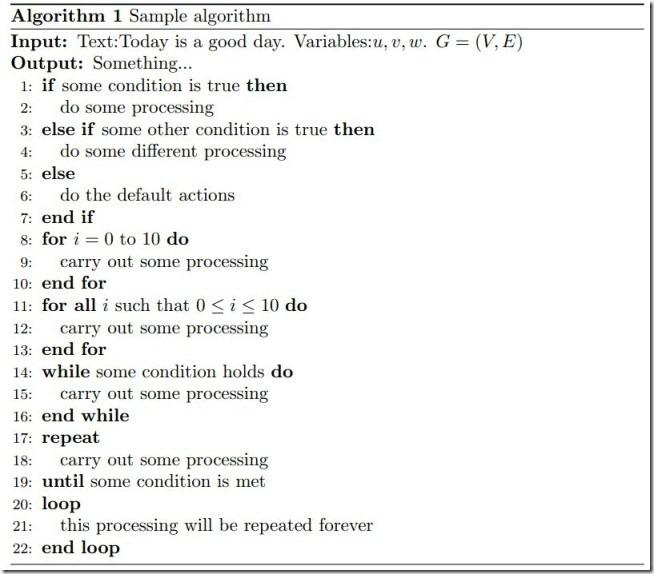\documentclass{article}

\usepackage{宏包}

\title{文章标题}

\author{作者}

\date{日期}

\begin{document}

\tableofcontents

\maketitle

\section{一级标题}
\subsection{二级标题}
\subsubsection{三级标题}

\paragraph{}

\par

\newpage

\end{document}

• 带有下标的符号需要保持下标直体（非斜体）：Tj×T_j \, \timesTj​× Tj✓T_{\rm j} \, \checkmarkTj​✓，可以用 {\rm ...} 将需要变为直体的部分括起来，如刚才的正确示范 Latex 表达式 为 T_{\rm j}
• 加粗显示文字：\textbf{文字内容} 【\bf{文字内容} 也可以达到加粗字体的效果，但 texStudio 不支持该表达式，使用后会使得后续文字全部加粗】
• 斜体显示文字：\textit{文字内容}
• 双引号：文字内''
• 当需要文字顶格时，通过 \noindent 达到文字顶格效果
• 使用 \ \hspace*{\fill} \par 可以勉强达到空一行的效果（下面会介绍更好的）

\usepackage{indentfirst}
\usepackage{setspace}
\usepackage[UTF8]{ctex}

\thispagestyle{}

\setcounter{page}{x}

\pagenumbering{}

\usepackage[a4paper,left=10mm,right=10mm,top=15mm,bottom=15mm]{geometry}

~\\

• 在 word 里的” 硬换行 “（一个向下的箭头）等同于 Latex 中”\\“ 这个换行符，意思是让文字换行但还是属于同一个段落
• 在 word 里的” 软换行 “（一个转弯的箭头）等同于 Latex 中”\par“ 这个段落标记或者直接空一行，意思是之后的文字就是另外一个段落。但是请注意，多个空行或\par连续只会被算作一个
• Latex 不像 word 那样有 “段前、段后、行间距” 等参数，只能通过设置 “段间距、行间距” 来控制排版，还可以像下面这样实现空行：

\newline
\vspace{2cm}
\\[2\baselikeskip]
\vspace{2\baselineskip}
\vspace{\fill}
复制代码
\begin{abstract}

\par\textbf{Keywords: } Keyword1; Keyword2; Keyword3
\end{abstract}

\begin{equation}

\end{equation}

\begin{align}

\end{align}

\newtheorem{definition}{\bf\it Definition \quad}[section]
\usepackage{amsthm}
\newtheorem*{parameter}{parameter}

\begin{parameter}
内容...
\end{parameter}

## 三线表格

\usepackage{booktabs}
\usepackage{diagbox}
\usepackage{multirow}

\begin{table}[htb]
\setlength{\abovecaptionskip}{0.0cm}
\setlength{\belowcaptionskip}{0.2cm}
\caption{table name}
\centering
\label{tab1}
\begin{tabular}{ccc}

\toprule
a & b & c \\
\midrule
1 & 2 & 3 \\
\bottomrule
\end{tabular}
\end{table}

## 合并多行的表格

\begin{table}
\caption{Multi}
\centering
\label{tab1}
\begin{tabular}{|c|c|c|c|c|}
\hline
\multirow{2}{*}{1} & 11 & 111 & 1111 & 11111 \\

\cline{2-5}

2 & 22 & 222 & 2222 & 22222 \\
\hline
3 & 33 & 333 & 3333 & 33333 \\
\hline
\end{tabular}
\end{table}• 合并多列只要将表达式 \multirow 改成 \multicolumn 即可

## 可以分行的表格

\newcommand{\tabincell}{\begin{tabular}{@{}#1@{}}#2\end{tabular}}

\begin{table*}[ht]
\caption{Title}
\centering
\begin{tabular}{|c|c|c|}
\toprule
{\bf Method} & {\bf 1} & {\bf 2} \\

\hline
\tabincell{c}{01\\02\\03}  & \tabincell{c}{11\\12}  & \tabincell{c}{21,\\22}  \\

\hline
11 & \tabincell{c}{dsads\\deawd} & \tabincell{c}{dewaf\\cewaef} \\
\bottomrule
\end{tabular}
\end{table*}## 带有斜线的表格

\begin{table}[ht]
\caption{example}
\centering
\begin{tabular}{|c|cc|}
\hline
\diagbox{bottom}{top} & A & B \\

\hline
1 & abc   & def \\
2 & ghijk & lmn \\
3 & opq   & rst \\
\hline
\end{tabular}
\end{table}• 插入跨栏表格只需要在 table 属性后面加上星号

\begin{table*}
...
\end{table*}
复制代码
• 当表格在文中显示过大或过小，可以添加

\setlength{\tabcolsep}{7mm}{
\begin{tabular}{lll}
...
\end{tabular}}
复制代码
• 当表格在文中显示过宽或过窄，可以添加

\resizebox{\textwidth}{7mm}{
\begin{tabular}{cc}
...
\end{tabular}}
复制代码
\usepackage{graphicx}
\usepackage[subfigure]{graphfig}

\begin{figure}
\centering
\includegraphics[height=4.5cm,width=7.5cm]{SVM_model.pdf}

\caption{This is a SVM model.}
\label{Fig1}
\end{figure}

• 插入跨栏图片只需要在 figure 属性后面加上星号

\begin{figure*}
...
\end{figure*}
复制代码
• 通常我们写论文插入图片的格式为 “eps，pdf，jpg”，这些格式都可以通过 matelab 编译之后把图片另存为就可以得到

• 当在 latex 中插入 eps 文件时，需要导入以下包

\usepackage{amsmath, amssymb, amsfonts, graphicx}
\usepackage{epstopdf}
复制代码
• 以下内容均是在 latex 中对图片的自定义设置

\setlength{\abovecaptionskip}{0cm}

\setlength{\belowcaptionskip}{-0.cm}

\scalebox{\includegraphics[trim={}, clip, width=, height=]}{图片的相对路径}
复制代码
• 在这里贴出一个链接，是在 latex 中插图和颜色的使用指导

## 通常框架

\usepackage{algorithm}
\usepackage{algorithmic}
\usepackage{amsmath}
\usepackage{xcolor}

\renewcommand{\algorithmicrequire}{\textbf{Input:}}
\renewcommand{\algorithmicensure}{\textbf{Output:}}

\begin{algorithm}
\caption{Sample algorithm}
\label{alg1}
\begin{algorithmic}
\REQUIRE Text:Today is a good day. Variables:$u,v,w$. $G=(V,E)$
\ENSURE Something...

\IF{some condition is true}
\STATE do some processing
\ELSIF{some other condition is true}
\STATE do some different processing
\ELSE
\STATE do the default actions
\ENDIF

\FOR{$i=0$ to $10$}
\STATE carry out some processing
\ENDFOR
\FORALL{$i$ such that $0\leq i\leq 10$}
\STATE carry out some processing
\ENDFOR

\WHILE{some condition holds}
\STATE carry out some processing
\ENDWHILE

\REPEAT
\STATE carry out some processing
\UNTIL{some condition is met}

\LOOP
\STATE this processing will be repeated forever
\ENDLOOP

\RETURN return parameters
\end{algorithmic}
\end{algorithm}\usepackage{algorithm}
\usepackage{algorithmicx}
\usepackage{algpseudocode}
\usepackage{amsmath}
\usepackage{xcolor}

\begin{algorithm}[htb]
\caption{sample algorithm}
\label{alg_label}
\begin{algorithmic}
\Require input paramters
\Ensure output
\Procedure{function name}{paramters}
\State Balabalabala...
\Statex Balabalabala...
\EndProcedure
\Return result.
\end{algorithmic}
\end{algorithm}

\usepackage{cite}

\cite{pa1}
\cite{pa1，pa2，pa3}

\bibliographystyle{plain}

\bibliographystyle{splncs04}

\bibliography{xxx}

Tip: 如果在前面已经生成了其它风格的 pdf，那么改代码之后直接编译会报错，这个时候只需要删除缓存文件. aux 和. bbl，再重新编译一遍就可以解决。当然碰上其它非语法错误的也可以采用这个方法解决。

1. plain，按字母的顺序排列，比较次序为作者、年度和标题；
2. unsrt，样式同 plain，只是按照引用的先后排序；
3. alpha，用作者名首字母 + 年份后两位作标号，以字母顺序排序；
4. abbrv，类似 plain，将月份全拼改为缩写，更显紧凑；
5. ieeetr，国际电气电子工程师协会期刊样式；
6. acm，美国计算机学会期刊样式；
7. siam，美国工业和应用数学学会期刊样式；
8. apalike，美国心理学学会期刊样式；
1. 血雨腥风霜：Latex 使用，插入标题...
2. ihoujie：latex 插入算法
3. 张一根：LaTeX 学习系列之 ---LaTeX 的总结
4. 碎金：LaTeX 排版入门的正确姿势（LaTeX 排版入门教程）
5. Liam Huang：一份其实很短的 LaTeX 入门文档
6. 无宠不惊过一生：LATEX 中常用的插图和插表格的方法
7. 刘海洋：《LaTex 入门》
8. LaTex 社区：社区首页
9. hzYang ：【开源项目】LaTeX 速查手册
10. MOON：LaTex 手册